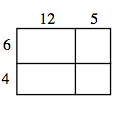### Home > CCAA8 > Chapter 7 Unit 3 > Lesson CC3: 7.1.2 > Problem7-20

7-20.Use the rectangle at right to answer the following questions. Homework Help ✎

1. Find the area of the entire rectangle. Explain how you found your solution.

The area of a figure is the same as the sum of the area of its parts.

$72+30+48+20$

2. Calculate the perimeter of the figure.

The perimeter of a figure is the sum of its sides.

$54$ units